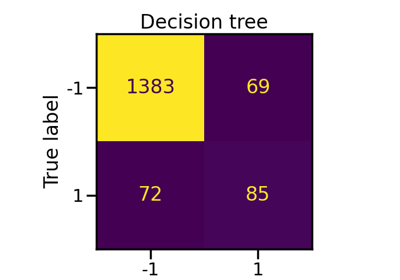# imblearn.ensemble.EasyEnsembleClassifier¶

class imblearn.ensemble.EasyEnsembleClassifier(n_estimators=10, base_estimator=None, *, warm_start=False, sampling_strategy='auto', replacement=False, n_jobs=None, random_state=None, verbose=0)[source]

Bag of balanced boosted learners also known as EasyEnsemble.

This algorithm is known as EasyEnsemble . The classifier is an ensemble of AdaBoost learners trained on different balanced boostrap samples. The balancing is achieved by random under-sampling.

Read more in the User Guide.

Parameters
n_estimatorsint, default=10

Number of AdaBoost learners in the ensemble.

The base AdaBoost classifier used in the inner ensemble. Note that you can set the number of inner learner by passing your own instance.

warm_startbool, default=False

When set to True, reuse the solution of the previous call to fit and add more estimators to the ensemble, otherwise, just fit a whole new ensemble.

sampling_strategyfloat, str, dict, callable, default=’auto’

Sampling information to sample the data set.

• When float, it corresponds to the desired ratio of the number of samples in the minority class over the number of samples in the majority class after resampling. Therefore, the ratio is expressed aswhereis the number of samples in the minority class andis the number of samples in the majority class after resampling.

Warning

float is only available for binary classification. An error is raised for multi-class classification.

• When str, specify the class targeted by the resampling. The number of samples in the different classes will be equalized. Possible choices are:

'majority': resample only the majority class;

'not minority': resample all classes but the minority class;

'not majority': resample all classes but the majority class;

'all': resample all classes;

'auto': equivalent to 'not minority'.

• When dict, the keys correspond to the targeted classes. The values correspond to the desired number of samples for each targeted class.

• When callable, function taking y and returns a dict. The keys correspond to the targeted classes. The values correspond to the desired number of samples for each class.

replacementbool, default=False

Whether or not to sample randomly with replacement or not.

n_jobsint, default=None

Number of CPU cores used during the cross-validation loop. None means 1 unless in a joblib.parallel_backend context. -1 means using all processors. See Glossary for more details.

random_stateint, RandomState instance, default=None

Control the randomization of the algorithm.

• If int, random_state is the seed used by the random number generator;

• If RandomState instance, random_state is the random number generator;

• If None, the random number generator is the RandomState instance used by np.random.

verboseint, optional (default=0)

Controls the verbosity of the building process.

BalancedBaggingClassifier

Bagging classifier for which each base estimator is trained on a balanced bootstrap.

BalancedRandomForestClassifier

Random forest applying random-under sampling to balance the different bootstraps.

RUSBoostClassifier

AdaBoost classifier were each bootstrap is balanced using random-under sampling at each round of boosting.

Notes

The method is described in .

Supports multi-class resampling by sampling each class independently.

References

1(1,2)

X. Y. Liu, J. Wu and Z. H. Zhou, “Exploratory Undersampling for Class-Imbalance Learning,” in IEEE Transactions on Systems, Man, and Cybernetics, Part B (Cybernetics), vol. 39, no. 2, pp. 539-550, April 2009.

Examples

>>> from collections import Counter
>>> from sklearn.datasets import make_classification
>>> from sklearn.model_selection import train_test_split
>>> from sklearn.metrics import confusion_matrix
>>> from imblearn.ensemble import EasyEnsembleClassifier
>>> X, y = make_classification(n_classes=2, class_sep=2,
... weights=[0.1, 0.9], n_informative=3, n_redundant=1, flip_y=0,
... n_features=20, n_clusters_per_class=1, n_samples=1000, random_state=10)
>>> print('Original dataset shape %s' % Counter(y))
Original dataset shape Counter({1: 900, 0: 100})
>>> X_train, X_test, y_train, y_test = train_test_split(X, y,
...                                                     random_state=0)
>>> eec = EasyEnsembleClassifier(random_state=42)
>>> eec.fit(X_train, y_train)
EasyEnsembleClassifier(...)
>>> y_pred = eec.predict(X_test)
>>> print(confusion_matrix(y_test, y_pred))
[[ 23   0]
[  2 225]]

Attributes
base_estimator_estimator

The base estimator from which the ensemble is grown.

estimators_list of estimators

The collection of fitted base estimators.

classes_array, shape (n_classes,)

The classes labels.

n_classes_int or list

The number of classes.

__init__(self, n_estimators=10, base_estimator=None, *, warm_start=False, sampling_strategy='auto', replacement=False, n_jobs=None, random_state=None, verbose=0)[source]

Initialize self. See help(type(self)) for accurate signature.

## Examples using imblearn.ensemble.EasyEnsembleClassifier¶